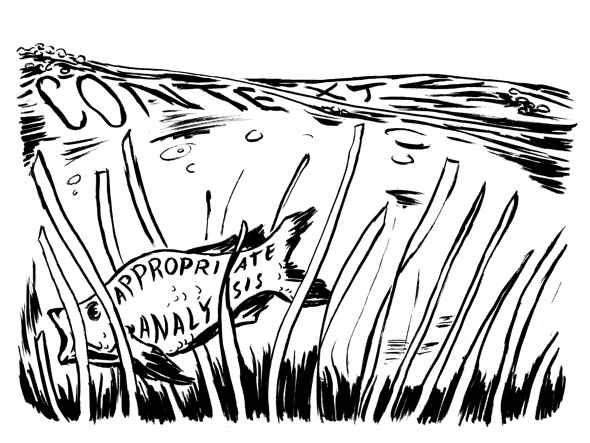## Statistical Significance

Tip: Do not report results as “approaching statistical significance”.

Rationale: Approaching statistical significance means that a result did not meet the predetermined level of significance, which is usually .05, but was close. In some ways it is a “nicer way of admitting that your results support the null hypothesis”.1 While it is tempting to report a result as approaching significance, it is problematic. A decision was made regarding the acceptable standard and now, based on the results, that decision is being modified to fit the data. This is a violation of the assumptions behind inferential statistics. The definition of “close” is problematic since it is based on what was found rather than any scientific rationale. If results are approaching significance it might be possible to collect data from more participants until the results are significant or until the significance gets worse. Another option would be to replicate the study.2

Tip: Limit the number of statistical tests that you do and have a rationale for each test that you do.

Rationale: Since statistical significance testing is based on a probability, the more statistical tests you do, the more likely you are to get statistically significant results that are incorrect.3 This cumulative error rate means that it is inappropriate to do 100 tests and then report as significant the five comparisons reaching the .05 level because of the dangers of capitalizing on chance. The major exception would be if the tests were intended to be exploratory and would be used only in a subsequent independent study to help generate hypotheses.

Tip: If statistical significance is found, check for effect size and, as needed, use a website that does effect size computations.4

Rationale: A finding of statistical significance means that the null hypotheses -- the hypotheses of no difference -- has been rejected. By itself, it does not mean that the findings are important or meaningful. It is important to determine the size or magnitude of the effects found. Effect sizes can be computed for group differences and for correlations.5 One well known effect size is Cohen's d. Cohen defined effect sizes as “small, d = .2,” “medium, d = .5,” and “large, d = .8,” but warned of the risks inherent in defining the terms in as “diverse a field of inquiry as behavioral science”.6 Larger effect sizes indicate that not only is a difference statistically significant but the likelihood is that the difference is meaningful as well.

Tip: Be sure that the statistical tests being used are the right ones for the data. This may involve using an online tool such as the one developed by the UCLA: Statistical Consulting Group7 or hiring a statistical consultant to provide assistance in the selection of appropriate tests.

Rationale: Data can be analyzed in multiple ways, each of which could yield legitimate answers. There are a number of factors that determine whether an analysis is appropriate including the number of dependent (or outcome) variables; the nature of the independent (or predictor) variables; whether your dependent variable is an interval variable, ordinal, or categorical variable8; and whether it is normally distributed.9 Use of an inappropriate statistic can lead to inaccurate results.

1 PSYCHblog (2012). Approaching significance.
2 Stassam (2012). Is the term “approaching significance” cheating?
3 Hopkins, W.G. (2000). A new view of statistics.
4 www.uccs.edu/~lbecker/
5 www.uccs.edu/lbecker/effect-size.html
6 Cohen, J. (1988). Statistical power analysis for the behavioral sciences (2nd ed.). Hillsdale, NJ: Lawrence Earlbaum Associates. p 25.
7 What statistical analysis should I use? UCLA: Statistical Consulting Group.
8 What is the difference between categorical, ordinal and interval variables? UCLA: Statistical Consulting Group.
9 What statistical analysis should I use? UCLA: Statistical Consulting Group.